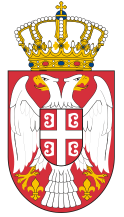Dokument se učitava

# Measurements ICourse code: 140707 | 6 ECTS credits

Basic information
Level of Studies: Undergraduate applied studies
Year of Study: 2
Semester: 4
Requirements: Knowledge of basic concepts of electrical engineering and electronics.
Goal: To provide basic theoretical and practical knowledge of the measurements in electrical engineering. To introduce students to properties of measuring devices and their application. To provide the students with the ability to practically realize procedures of measuring the basic electrical quantities and ability to use the computer for processing and analysis of measurement data.
Outcome: At the end of the course, the students will be familiar with the basic principles of measurement techniques, structures and working methods of measuring electrical circuits. The students will be able to work with modern electronic measuring instruments. The students will be able to use computers for mathematical processing and graphical display of measurement results.
Contents of the course
Theoretical instruction:
1. Measurements, and metrology (significance, historical background). Basic concepts and definitions.
2. Physical quantities and units of measurement.
3. Measurements (measurement methodology, measurement model, the results of measurements).
4. Measurement error (error term, classification, measurement uncertainty, the account of errors).
5. Measuring instruments and their characteristics. Laboratory standards.
6. Analog elements of electronic measuring instruments (operational amplifiers, voltage reference sources).
7. Digital elements of electronic measuring instruments. Logic gates. Counters and registers.
8. Quantization and coding. Methods of implementation.
9. Measurements of time intervals and a frequency.
10. Analog-to-digital converters.
11. Basic concepts of measurement in electrical engineering.
12. Measurement of DC voltage and DC current.
13. Measurement of electrical resistance.
14. Legislative metrology.
15. Measuring the output resistance of the current source.
Practical instruction (Problem solving sessions/Lab work/Practical training):
1. Practical training program follows the lectures.
Textbooks and References
1. P. Bošnjaković, Umeće merenja, VIŠER, 2011.
2. D. Prokin, P. Bošnjaković , Industrijska metrologija, VIŠER, 2016.
3. P.Bošnjaković, Specijalna elektronska kola, VIŠER, 2013.
4. P. Bošnjaković, D. Prokin, Merenja 1, Priručnik za laboratorijske vežbe, VIŠER, 2018.
5. J. Webster, The measurement, instrumentation, and sensors handbook, IEEE Press, 1999.
Number of active classes (weekly)
Lectures: 3
Practical classes: 2
Other types of classes: 0
Grading (maximum number of points: 100)
Pre-exam obligations
Points
activities during lectures
15
activities on practial excersises
15
seminary work
0
colloquium
40
Final exam
Points
Written exam
30
Oral exam
0

#### Associate